# RS Aggarwal Solutions for Class 8 Maths Chapter 7 - Factorisation

RS Aggarwal Solutions for Class 8 Maths Chapter 7- Factorisation, are provided here. Our expert faculty team has prepared solutions to help you with your exam preparation to acquire good marks in Maths. RS Aggarwal Solutions for Class 8 Maths comes in very handy at this point. Our solution module utilises various shortcut tips and practical examples to explain all the exercise questions in a simple and easily understandable language. If you wish to secure an excellent score, solving RS Aggarwal Class 8 Solutions is an utmost necessity. These solutions will help you in gaining knowledge and strong command over the subject. Practising the textbook questions will help you in analysing your level of preparation and understanding of the concept.

To help you understand and solve the problems, we at BYJU’S have designed these solutions in a very easy and understandable manner that helps students solve problems in the most efficient ways possible. The RS Aggarwal Solutions for Class 8 Chapter 7 is available on this page where solutions are solved in detail. Download pdf of Class 8 Chapter 7 in their respective links.

## Download PDF of RS Aggarwal Solutions for Class 8 Maths Chapter 7 – Factorisation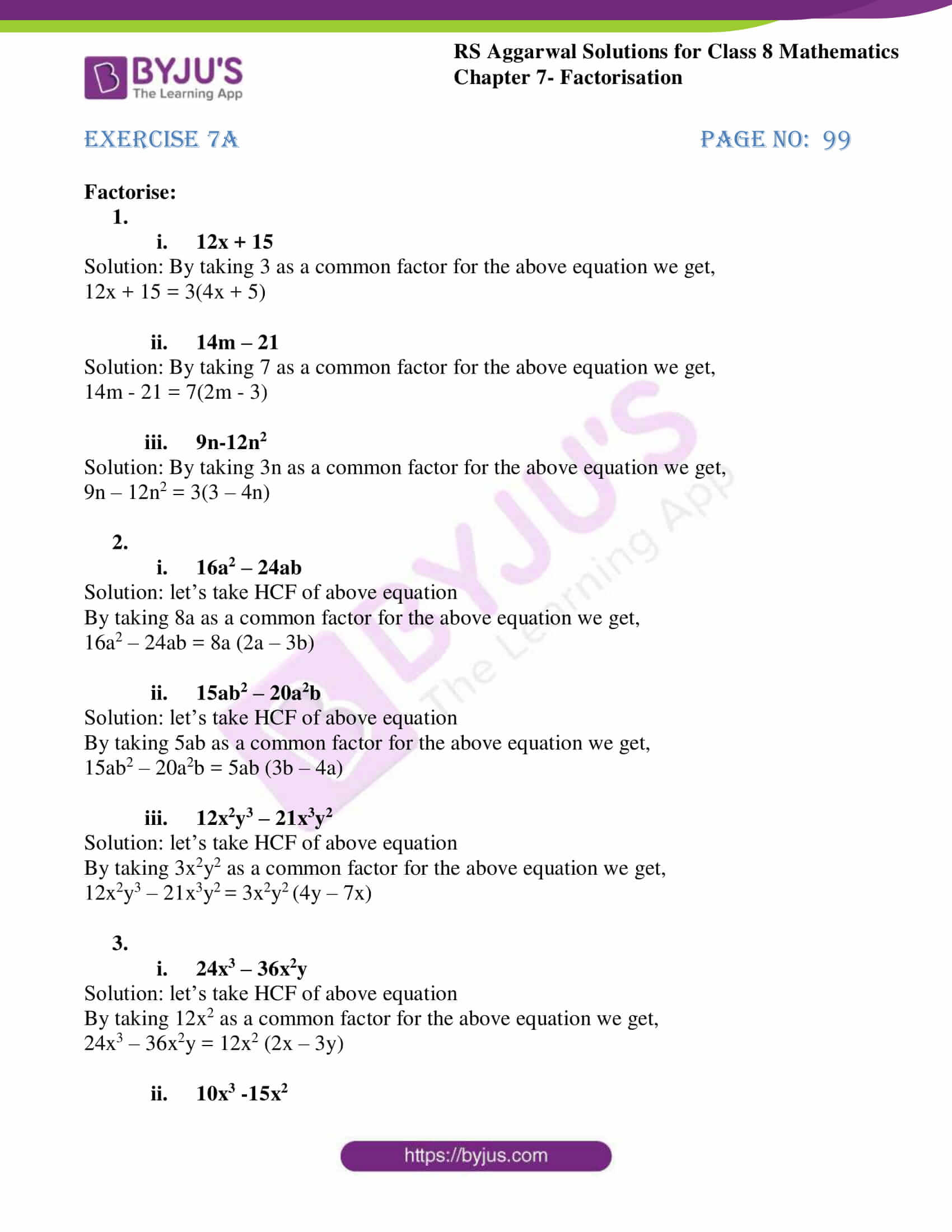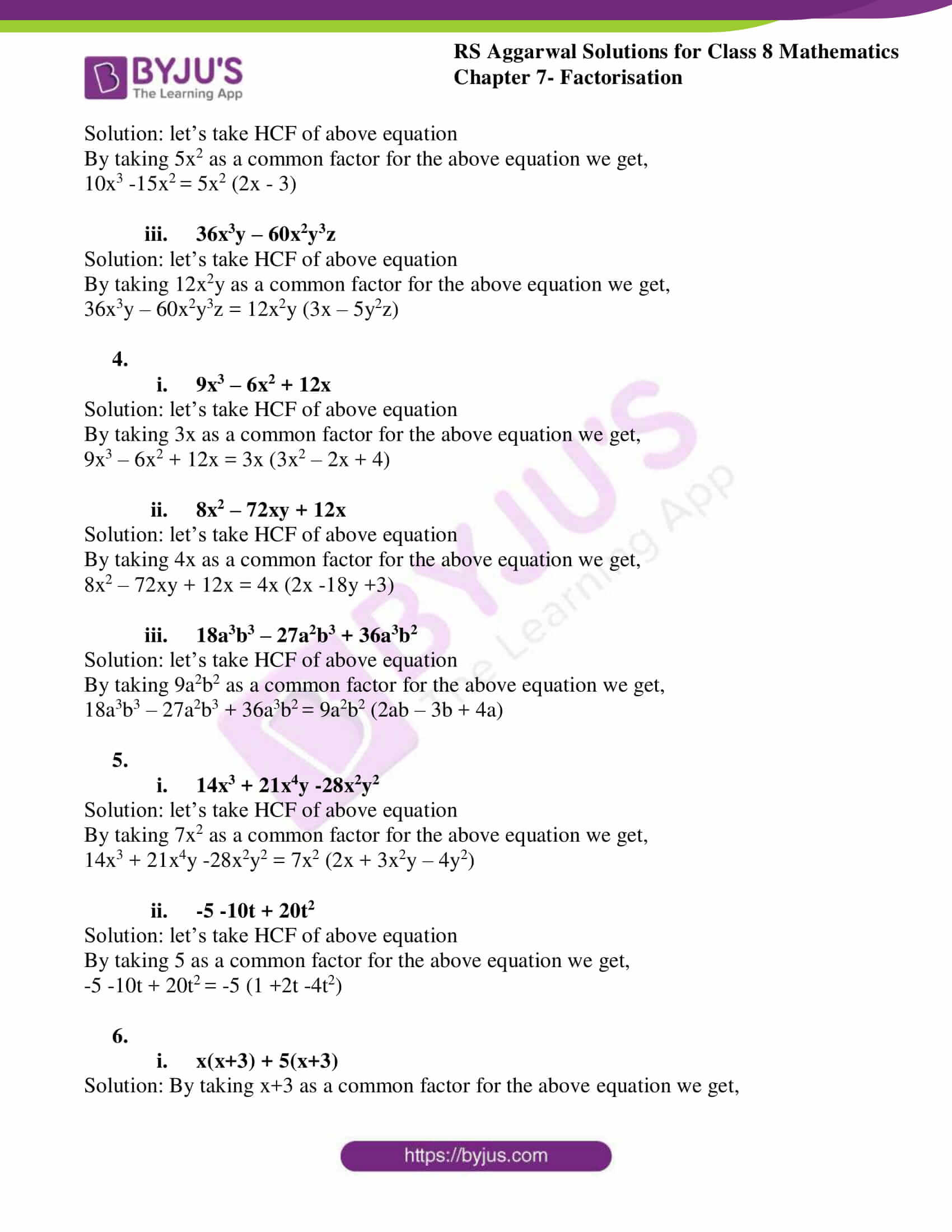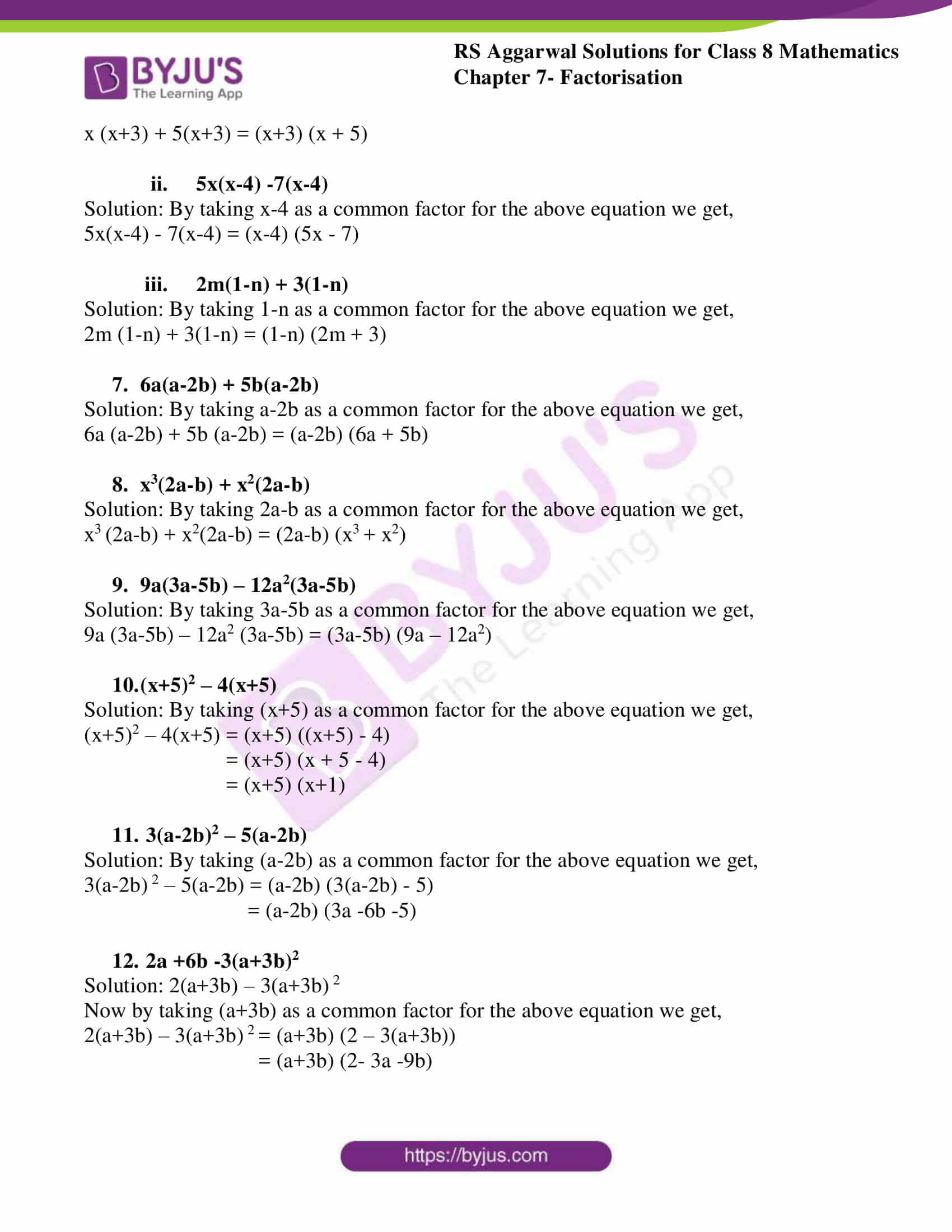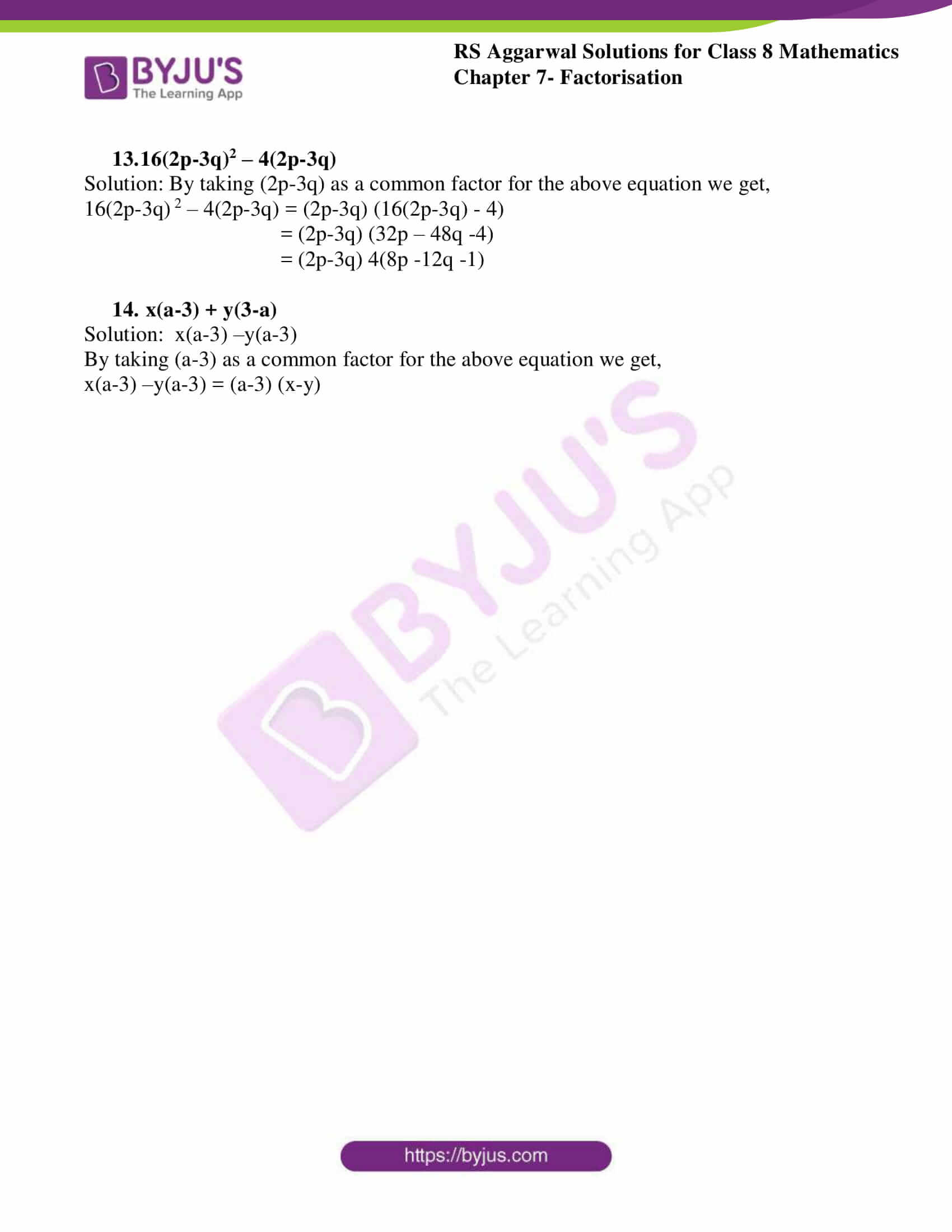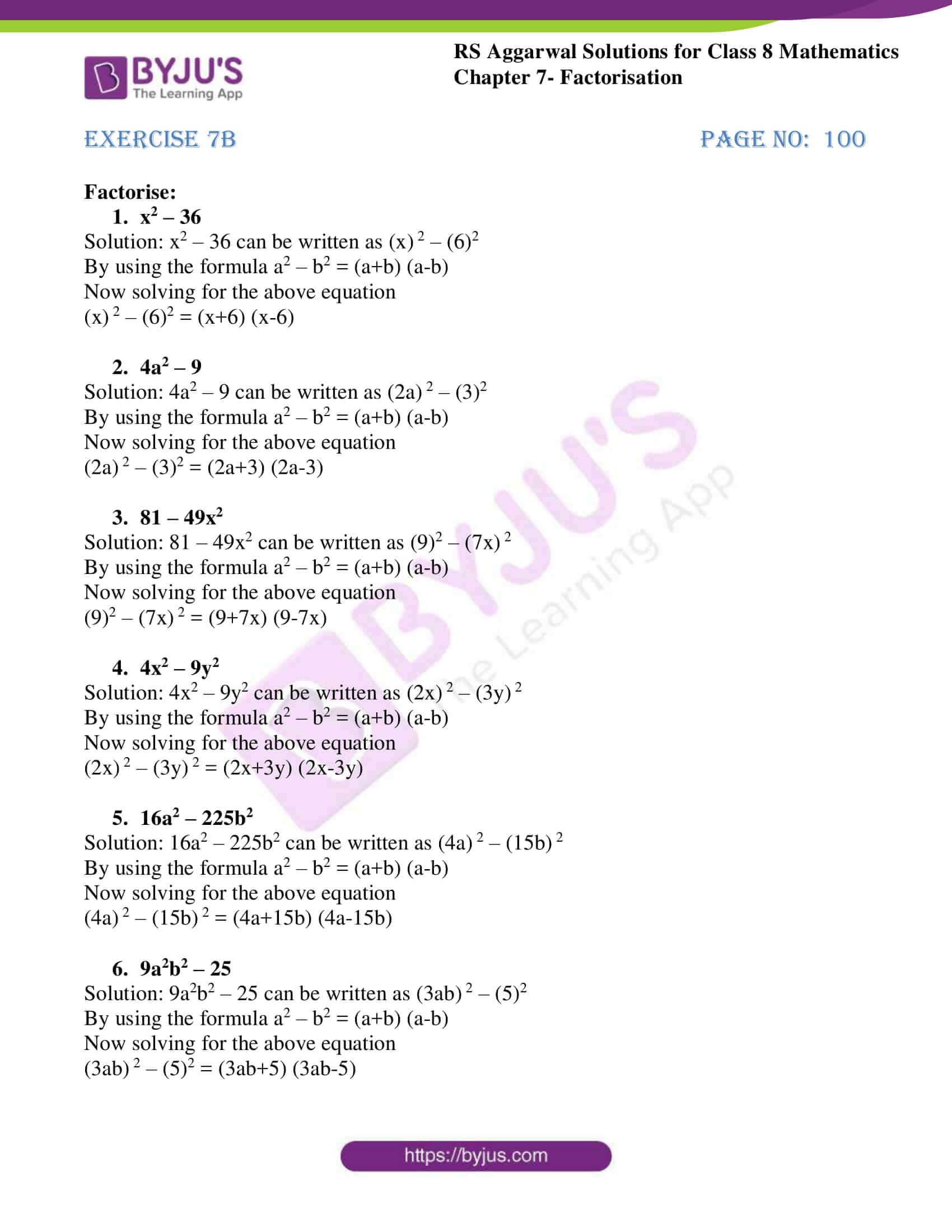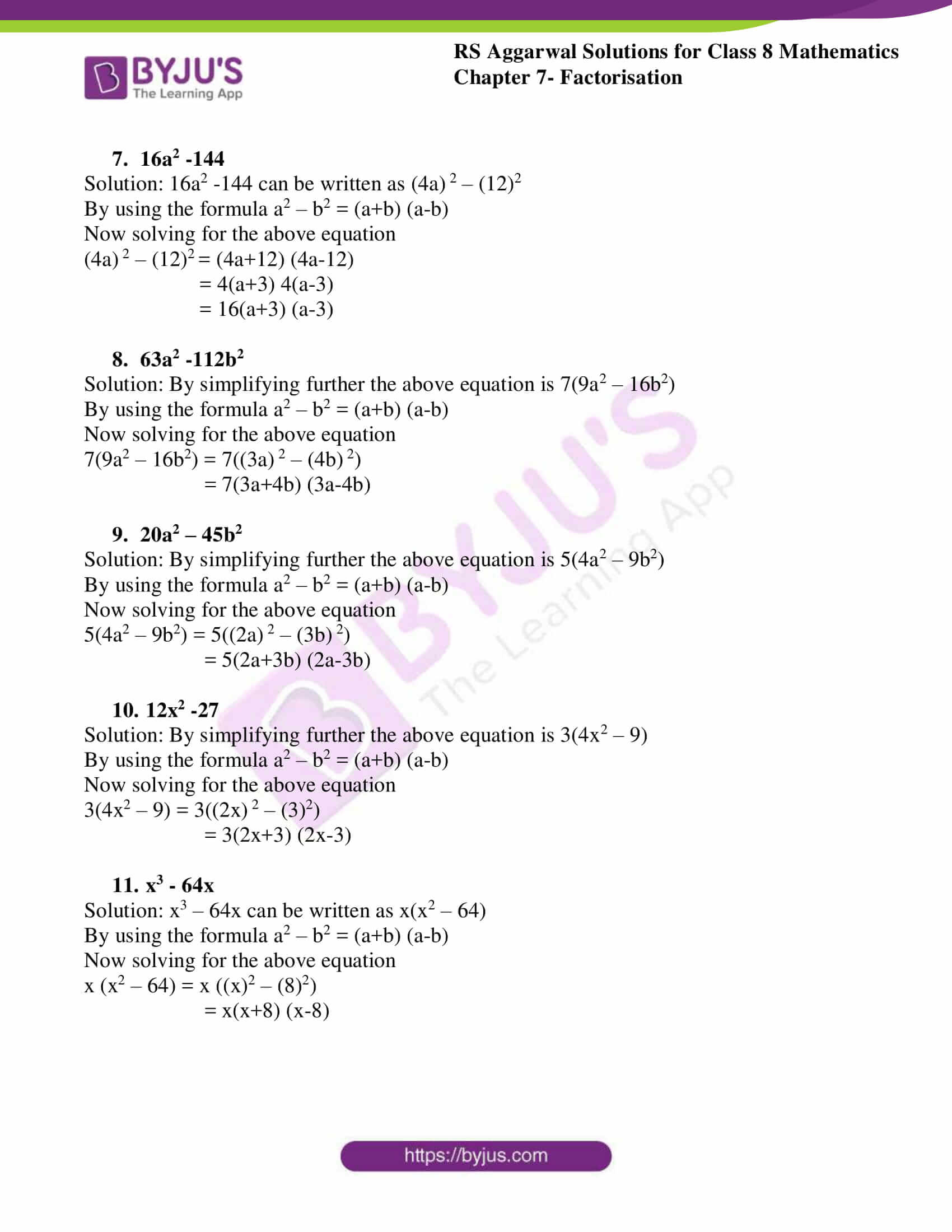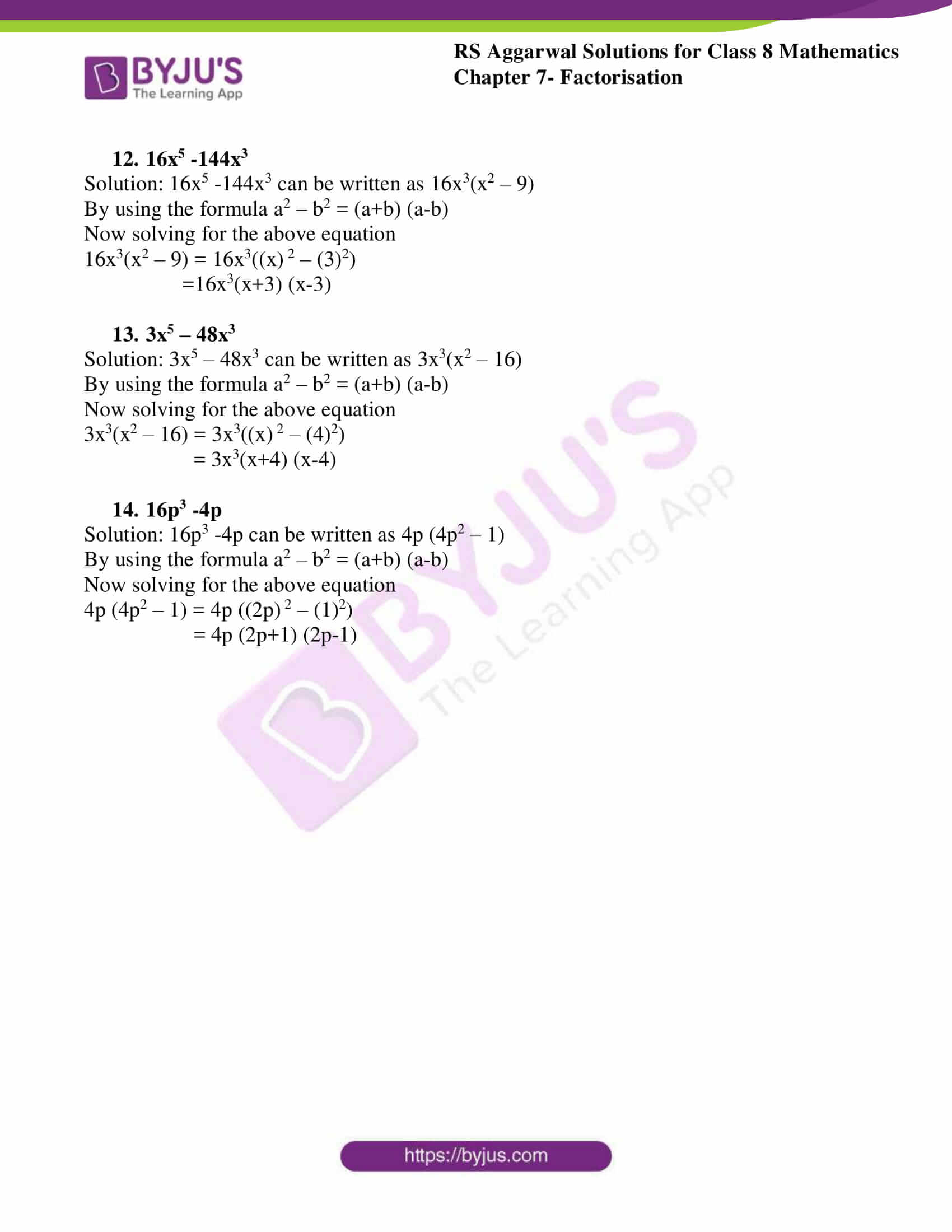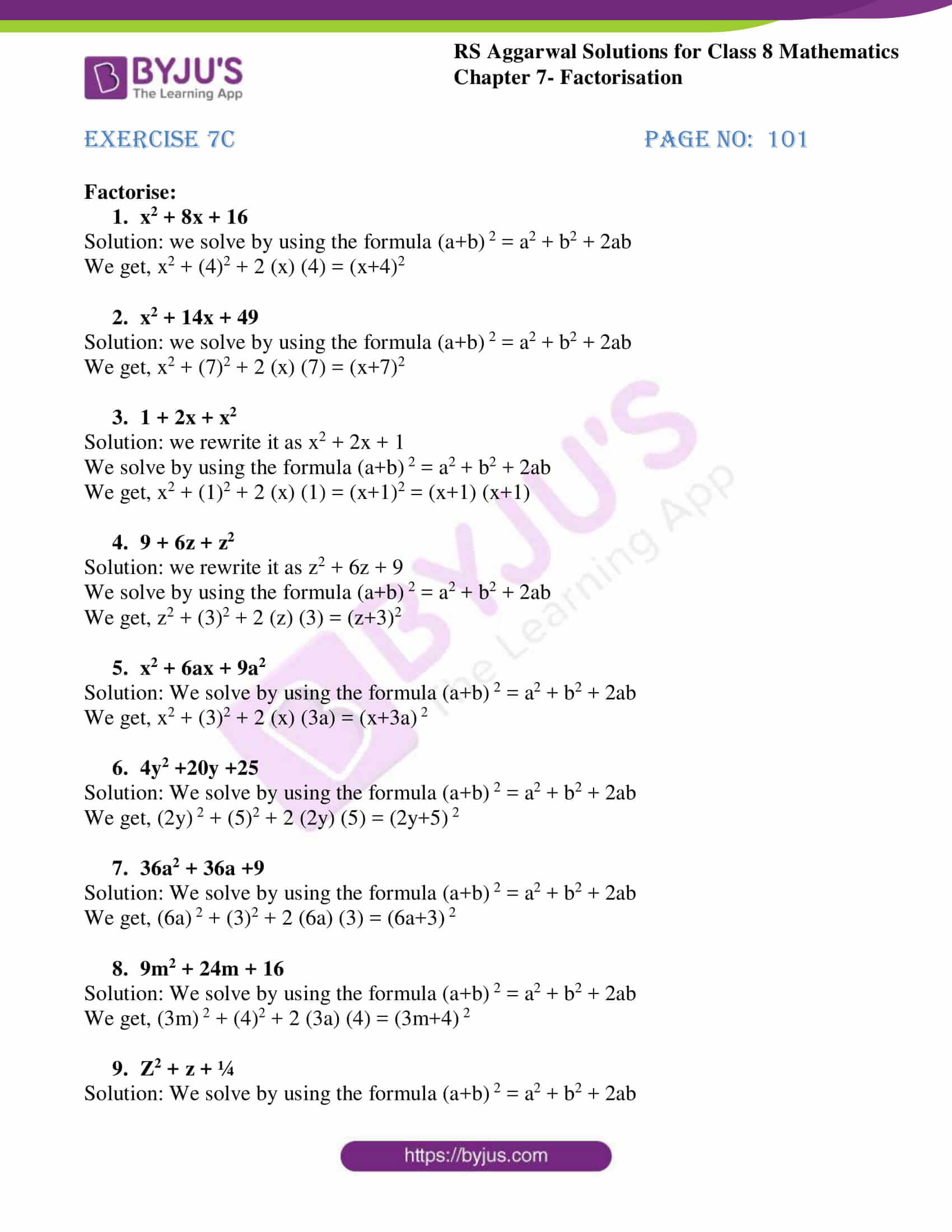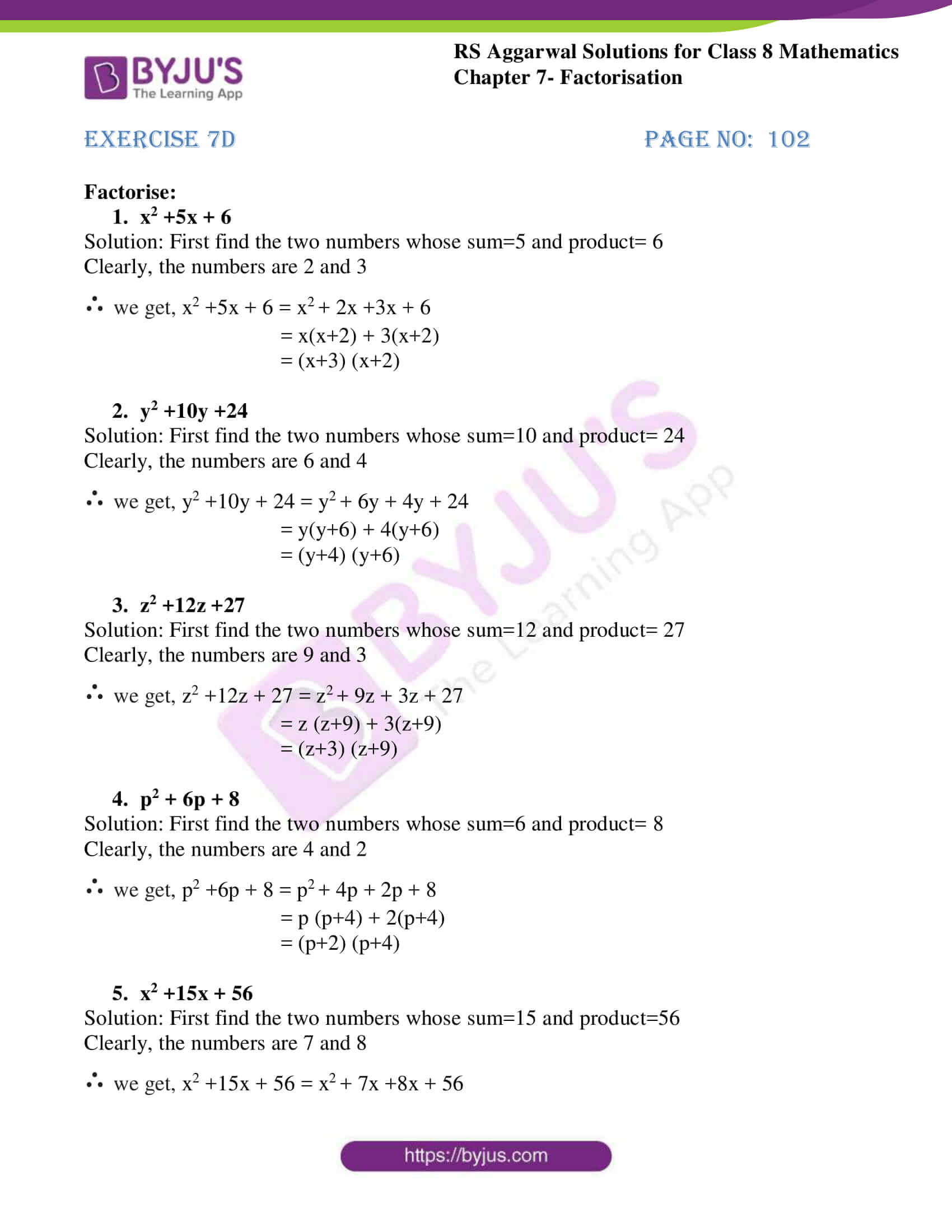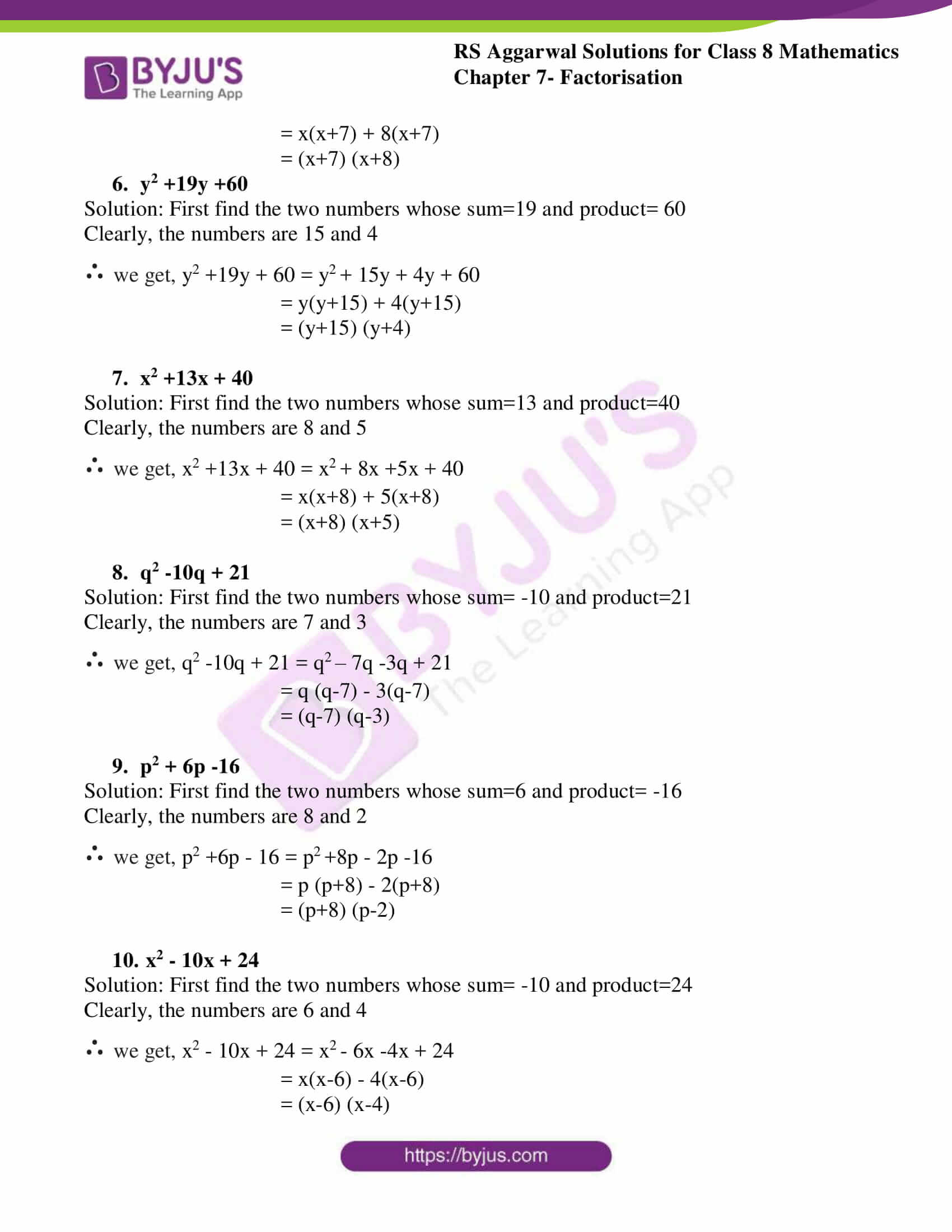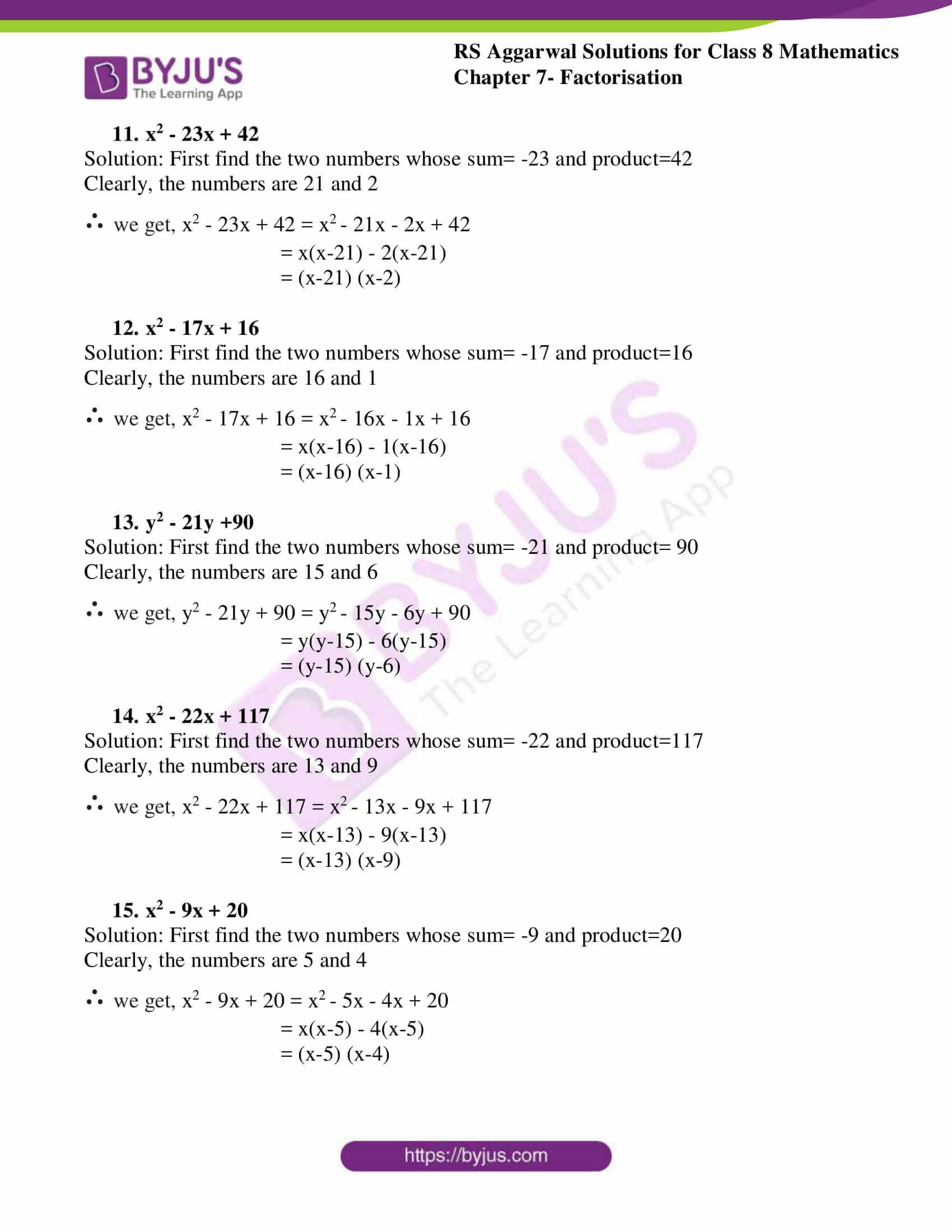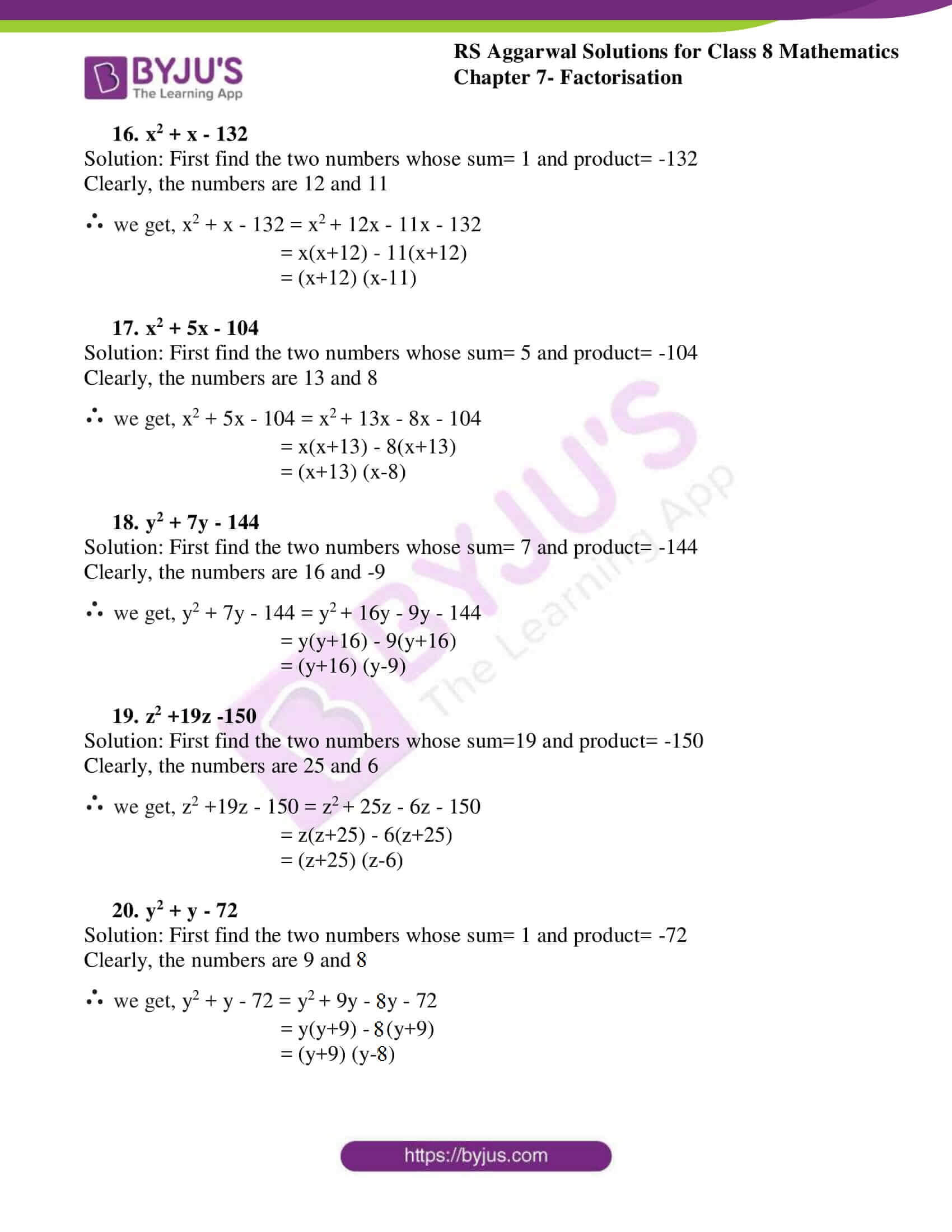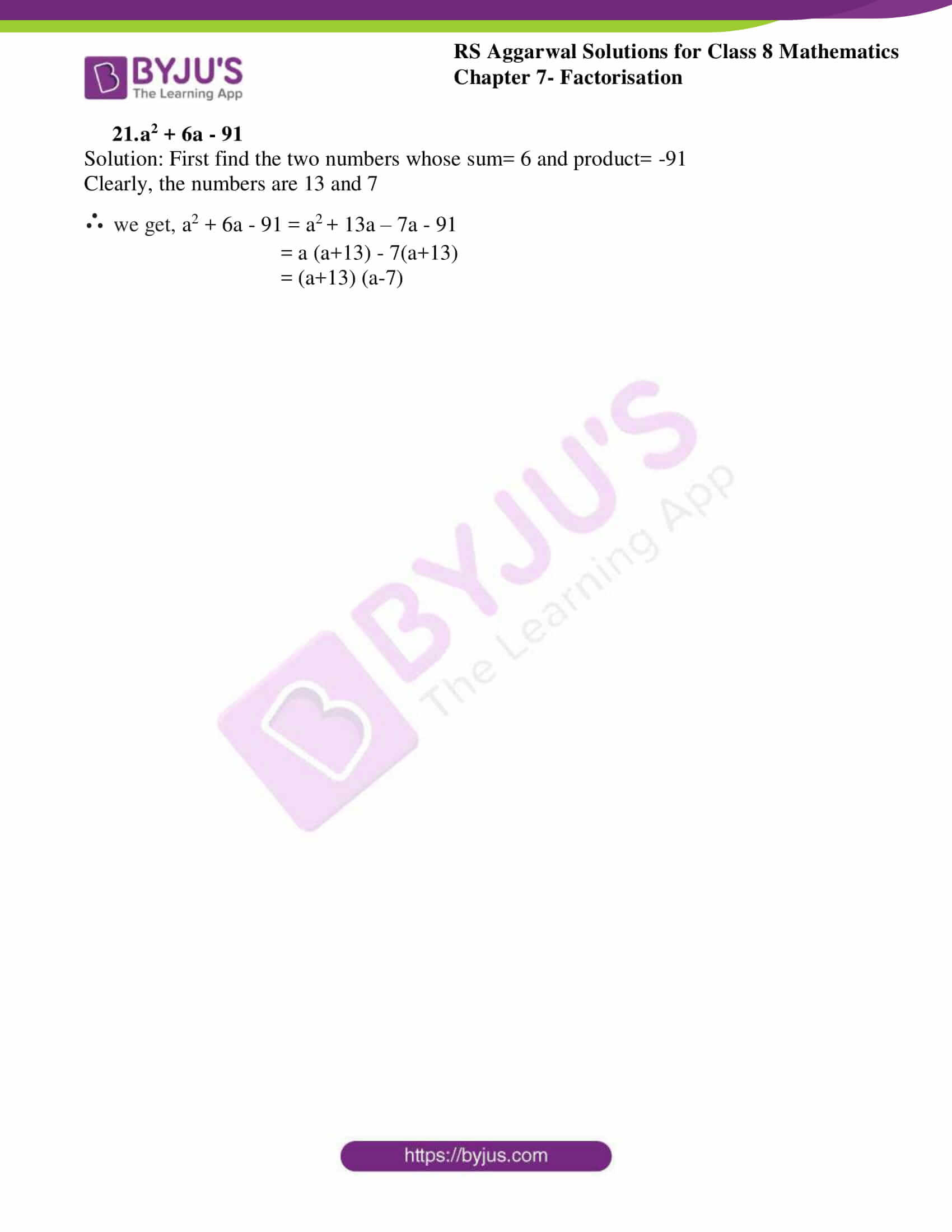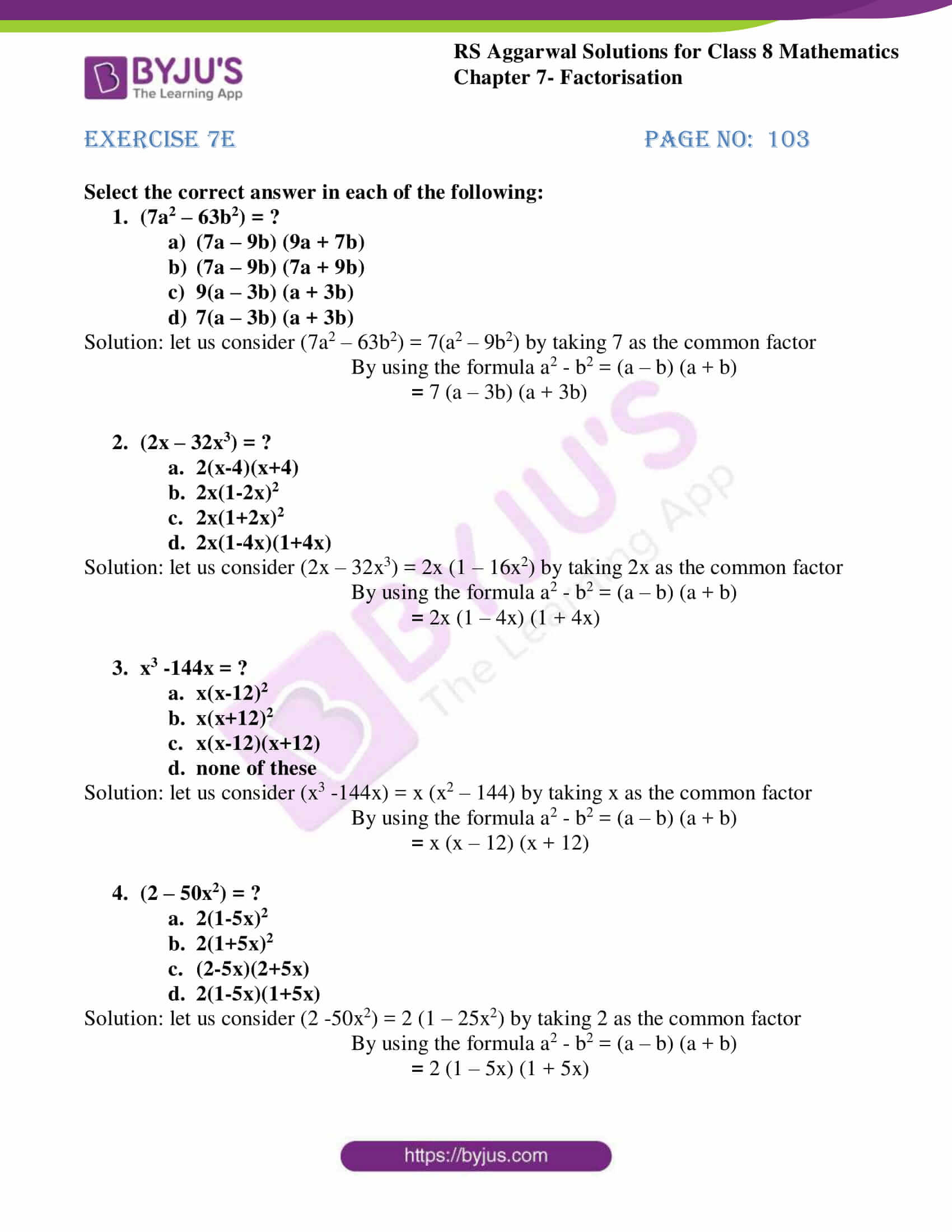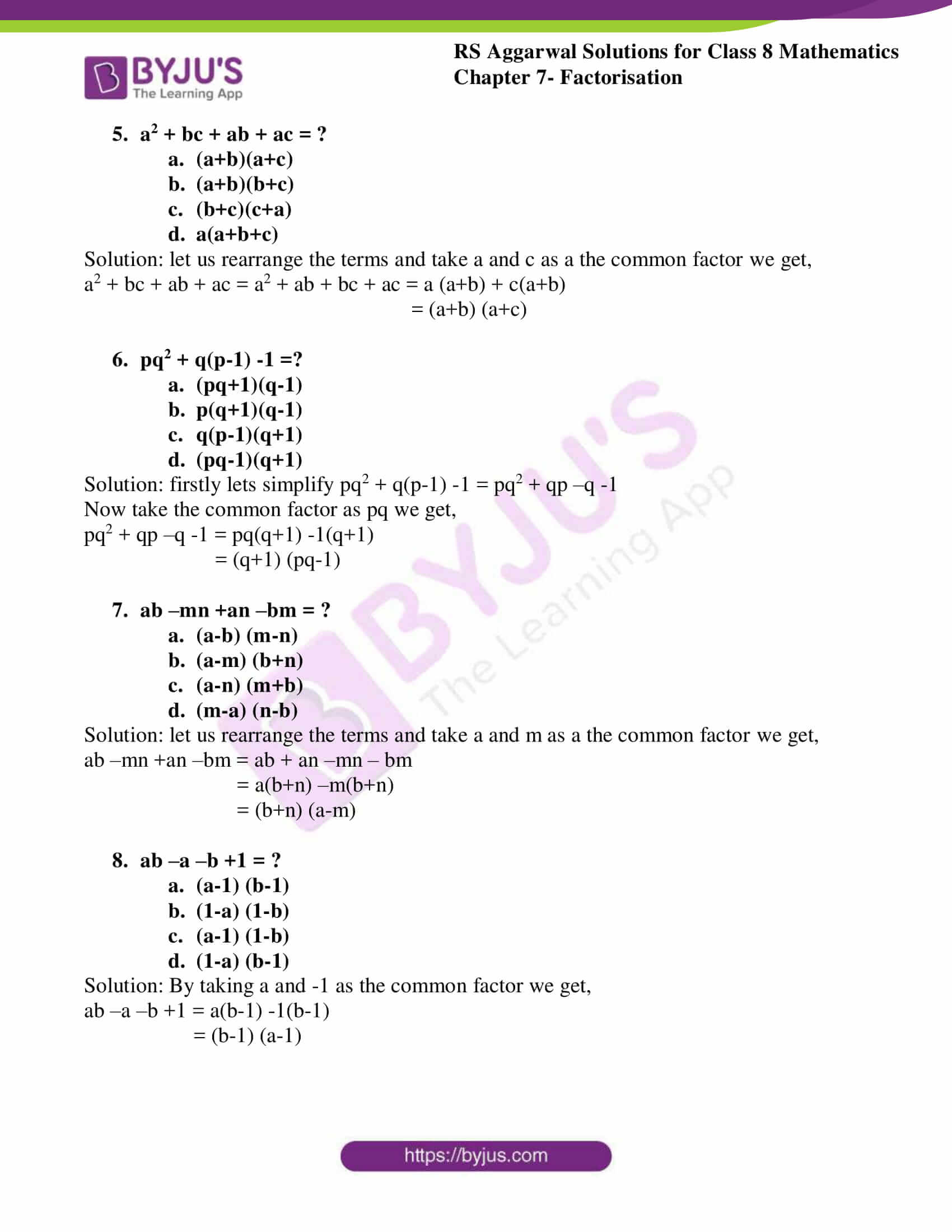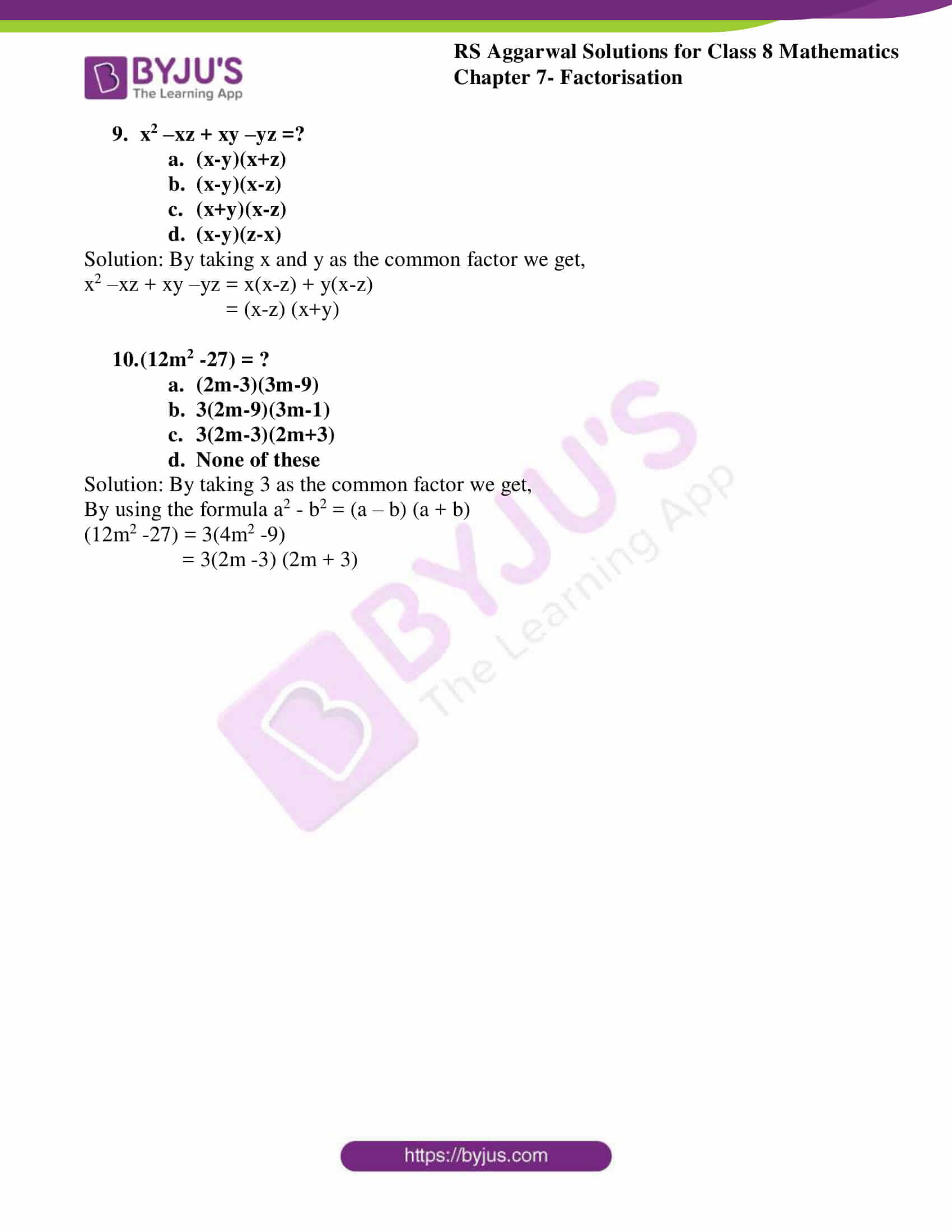## Exercise 7A

Factorise:

1.i 12x + 15

Solution:

By taking 3 as a common factor for the above equation we get,

12x + 15 = 3(4x + 5)

ii 14m – 21

Solution:

By taking 7 as a common factor for the above equation we get,

14m – 21 = 7(2m – 3)

iii 9n-12n2

Solution:

By taking 3n as a common factor for the above equation we get,

9n – 12n2 = 3(3 – 4n)

2.

i 16a2 – 24ab

Solution:

let’s take HCF of above equation

By taking 8a as a common factor for the above equation we get,

16a2 – 24ab = 8a (2a – 3b)

ii 15ab2 – 20a2b

Solution:

let’s take HCF of above equation

By taking 5ab as a common factor for the above equation we get,

15ab2 – 20a2b = 5ab (3b – 4a)

iii 12x2y3 – 21x3y2

Solution:

let’s take HCF of above equation

By taking 3x2y2 as a common factor for the above equation we get,

12x2y3 – 21x3y2 = 3x2y2 (4y – 7x)

3.

i 24x3 – 36x2y

Solution:

let’s take HCF of above equation

By taking 12x2 as a common factor for the above equation we get,

24x3 – 36x2y = 12x2 (2x – 3y)

ii 10x3 -15x2

Solution:

let’s take HCF of above equation

By taking 5x2 as a common factor for the above equation we get,

10x3 -15x2 = 5x2 (2x – 3)

iii 36x3y – 60x2y3z

Solution:

let’s take HCF of above equation

By taking 12x2y as a common factor for the above equation we get,

36x3y – 60x2y3z = 12x2y (3x – 5y2z)

4.

i 9x3 – 6x2 + 12x

Solution:

let’s take HCF of above equation

By taking 3x as a common factor for the above equation we get,

9x3 – 6x2 + 12x = 3x (3x2 – 2x + 4)

ii 8x2 – 72xy + 12x

Solution:

let’s take HCF of above equation

By taking 4x as a common factor for the above equation we get,

8x2 – 72xy + 12x = 4x (2x -18y +3)

iii 18a3b3 – 27a2b3 + 36a3b2

Solution:

let’s take HCF of above equation

By taking 9a2b2 as a common factor for the above equation we get,

18a3b3 – 27a2b3 + 36a3b2 = 9a2b2 (2ab – 3b + 4a)

5.

i 14x3 + 21x4y -28x2y2

Solution:

let’s take HCF of above equation

By taking 7x2 as a common factor for the above equation we get,

14x3 + 21x4y -28x2y2 = 7x2 (2x + 3x2y – 4y2)

ii -5 -10t + 20t2

Solution:

let’s take HCF of above equation

By taking 5 as a common factor for the above equation we get,

-5 -10t + 20t2 = -5 (1 +2t -4t2)

6.

i x(x+3) + 5(x+3)

Solution:

By taking x+3 as a common factor for the above equation we get,

x (x+3) + 5(x+3) = (x+3) (x + 5)

ii 5x(x-4) -7(x-4)

Solution:

By taking x-4 as a common factor for the above equation we get,

5x(x-4) – 7(x-4) = (x-4) (5x – 7)

iii 2m(1-n) + 3(1-n)

Solution:

By taking 1-n as a common factor for the above equation we get,

2m (1-n) + 3(1-n) = (1-n) (2m + 3)

7. 6a(a-2b) + 5b(a-2b)

Solution: By taking a-2b as a common factor for the above equation we get,

6a (a-2b) + 5b (a-2b) = (a-2b) (6a + 5b)

8. x3(2a-b) + x2(2a-b)

Solution: By taking 2a-b as a common factor for the above equation we get,

x3 (2a-b) + x2(2a-b) = (2a-b) (x3 + x2)

9. 9a(3a-5b) – 12a2(3a-5b)

Solution: By taking 3a-5b as a common factor for the above equation we get,

9a (3a-5b) – 12a2 (3a-5b) = (3a-5b) (9a – 12a2)

10. (x+5)2 – 4(x+5)

Solution: By taking (x+5) as a common factor for the above equation we get,

(x+5)2 – 4(x+5) = (x+5) ((x+5) – 4)

= (x+5) (x + 5 – 4)

= (x+5) (x+1)

11. 3(a-2b)2 – 5(a-2b)

Solution: By taking (a-2b) as a common factor for the above equation we get,

3(a-2b) 2 – 5(a-2b) = (a-2b) (3(a-2b) – 5)

= (a-2b) (3a -6b -5)

12. 2a +6b -3(a+3b)2

Solution: 2(a+3b) – 3(a+3b) 2

Now by taking (a+3b) as a common factor for the above equation we get,

2(a+3b) – 3(a+3b) 2 = (a+3b) (2 – 3(a+3b))

= (a+3b) (2- 3a -9b)

13. 16(2p-3q)2 – 4(2p-3q)

Solution: By taking (2p-3q) as a common factor for the above equation we get,

16(2p-3q) 2 – 4(2p-3q) = (2p-3q) (16(2p-3q) – 4)

= (2p-3q) (32p – 48q -4)

= (2p-3q) 4(8p -12q -1)

14. x(a-3) + y(3-a)

Solution: x(a-3) –y(a-3)

By taking (a-3) as a common factor for the above equation we get,

x(a-3) –y(a-3) = (a-3) (x-y)

## Exercise 7B

Factorise:

1. x2 – 36

Solution:

x2 – 36 can be written as (x) 2 – (6)2

By using the formula a2 – b2 = (a+b) (a-b)

Now solving for the above equation

(x) 2 – (6)2 = (x+6) (x-6)

2. 4a2 – 9

Solution:

4a2 – 9 can be written as (2a) 2 – (3)2

By using the formula a2 – b2 = (a+b) (a-b)

Now solving for the above equation

(2a) 2 – (3)2 = (2a+3) (2a-3)

3. 81 – 49x2

Solution:

81 – 49x2 can be written as (9)2 – (7x) 2

By using the formula a2 – b2 = (a+b) (a-b)

Now solving for the above equation

(9)2 – (7x) 2 = (9+7x) (9-7x)

4. 4x2 – 9y2

Solution:

4x2 – 9y2 can be written as (2x) 2 – (3y) 2

By using the formula a2 – b2 = (a+b) (a-b)

Now solving for the above equation

(2x) 2 – (3y) 2 = (2x+3y) (2x-3y)

5. 16a2 – 225b2

Solution:

16a2 – 225b2 can be written as (4a) 2 – (15b) 2

By using the formula a2 – b2 = (a+b) (a-b)

Now solving for the above equation

(4a) 2 – (15b) 2 = (4a+15b) (4a-15b)

6. 9a2b2 – 25

Solution:

9a2b2 – 25 can be written as (3ab) 2 – (5)2

By using the formula a2 – b2 = (a+b) (a-b)

Now solving for the above equation

(3ab) 2 – (5)2 = (3ab+5) (3ab-5)

7. 16a2 -144

Solution:

16a2 -144 can be written as (4a) 2 – (12)2

By using the formula a2 – b2 = (a+b) (a-b)

Now solving for the above equation

(4a) 2 – (12)2 = (4a+12) (4a-12)= 4(a+3) 4(a-3)= 16(a+3) (a-3)

8. 63a2 -112b2

Solution:

By simplifying further the above equation is 7(9a2 – 16b2)

By using the formula a2 – b2 = (a+b) (a-b)

Now solving for the above equation

7(9a2 – 16b2) = 7((3a) 2 – (4b) 2)= 7(3a+4b) (3a-4b)

9. 20a2 – 45b2

Solution:

By simplifying further the above equation is 5(4a2 – 9b2)

By using the formula a2 – b2 = (a+b) (a-b)

Now solving for the above equation

5(4a2 – 9b2) = 5((2a) 2 – (3b) 2)= 5(2a+3b) (2a-3b)

10. 12x2 -27

Solution:

By simplifying further the above equation is 3(4x2 – 9)

By using the formula a2 – b2 = (a+b) (a-b)

Now solving for the above equation

3(4x2 – 9) = 3((2x) 2 – (3)2)= 3(2x+3) (2x-3)

11. x3 – 64x

Solution:

x3 – 64x can be written as x(x2 – 64)

By using the formula a2 – b2 = (a+b) (a-b)

Now solving for the above equation

x (x2 – 64) = x ((x)2 – (8)2)= x(x+8) (x-8)

12. 16x5 -144x3

Solution:

16x5 -144x3 can be written as 16x3(x2 – 9)

By using the formula a2 – b2 = (a+b) (a-b)

Now solving for the above equation

16x3(x2 – 9) = 16x3((x) 2 – (3)2)=16x3(x+3) (x-3)

13. 3x5 – 48x3

Solution:

3x5 – 48x3 can be written as 3x3(x2 – 16)

By using the formula a2 – b2 = (a+b) (a-b)

Now solving for the above equation

3x3(x2 – 16) = 3x3((x) 2 – (4)2)= 3x3(x+4) (x-4)

14. 16p3 -4p

Solution:

16p3 -4p can be written as 4p (4p2 – 1)

By using the formula a2 – b2 = (a+b) (a-b)

Now solving for the above equation

4p (4p2 – 1) = 4p ((2p) 2 – (1)2)= 4p (2p+1) (2p-1)

## Exercise 7C

Factorise:

1. x2 + 8x + 16

Solution:

we solve by using the formula (a+b) 2 = a2 + b2 + 2ab

We get, x2 + (4)2 + 2 (x) (4) = (x+4)2

2. x2 + 14x + 49

Solution:

we solve by using the formula (a+b) 2 = a2 + b2 + 2ab

We get, x2 + (7)2 + 2 (x) (7) = (x+7)2

3. 1 + 2x + x2

Solution:

we rewrite it as x2 + 2x + 1

We solve by using the formula (a+b) 2 = a2 + b2 + 2ab

We get, x2 + (1)2 + 2 (x) (1) = (x+1)2 = (x+1) (x+1)

4. 9 + 6z + z2

Solution:

we rewrite it as z2 + 6z + 9

We solve by using the formula (a+b) 2 = a2 + b2 + 2ab

We get, z2 + (3)2 + 2 (z) (3) = (z+3)2

5. x2 + 6ax + 9a2

Solution:

We solve by using the formula (a+b) 2 = a2 + b2 + 2ab

We get, x2 + (3)2 + 2 (x) (3a) = (x+3a) 2

6. 4y2 +20y +25

Solution:

We solve by using the formula (a+b) 2 = a2 + b2 + 2ab

We get, (2y) 2 + (5)2 + 2 (2y) (5) = (2y+5) 2

7. 36a2 + 36a +9

Solution:

We solve by using the formula (a+b) 2 = a2 + b2 + 2ab

We get, (6a) 2 + (3)2 + 2 (6a) (3) = (6a+3) 2

8. 9m2 + 24m + 16

Solution:

We solve by using the formula (a+b) 2 = a2 + b2 + 2ab

We get, (3m) 2 + (4)2 + 2 (3a) (4) = (3m+4) 2

9. z2 + z + ¼

Solution:

We solve by using the formula (a+b) 2 = a2 + b2 + 2ab

We get, (z) 2 + (1/2)2 + 2 (z) (1/2) = (z+1/2) 2

## Exercise 7D

Factorise:

1. x2 +5x + 6

Solution:

First find the two numbers whose sum=5 and product= 6

Clearly, the numbers are 2 and 3

∴ we get, x2 +5x + 6 = x2 + 2x +3x + 6= x(x+2) + 3(x+2)= (x+3) (x+2)

2. y2 +10y +24

Solution:

First find the two numbers whose sum=10 and product= 24

Clearly, the numbers are 6 and 4

∴ we get, y2 +10y + 24 = y2 + 6y + 4y + 24= y(y+6) + 4(y+6)= (y+4) (y+6)

3. z2 +12z +27

Solution:

First find the two numbers whose sum=12 and product= 27

Clearly, the numbers are 9 and 3

∴ we get, z2 +12z + 27 = z2 + 9z + 3z + 27= z (z+9) + 3(z+9)= (z+3) (z+9)

4. p2 + 6p + 8

Solution:

First find the two numbers whose sum=6 and product= 8

Clearly, the numbers are 4 and 2

∴ we get, p2 +6p + 8 = p2 + 4p + 2p + 8= p (p+4) + 2(p+4)= (p+2) (p+4)

5. x2 +15x + 56

Solution:

First find the two numbers whose sum=15 and product=56

Clearly, the numbers are 7 and 8

∴ we get, x2 +15x + 56 = x2 + 7x +8x + 56= x(x+7) + 8(x+7)= (x+7) (x+8)

6. y2 +19y +60

Solution:

First find the two numbers whose sum=19 and product= 60

Clearly, the numbers are 15 and 4

∴ we get, y2 +19y + 60 = y2 + 15y + 4y + 60= y(y+15) + 4(y+15)= (y+15) (y+4)

7. x2 +13x + 40

Solution:

First find the two numbers whose sum=13 and product=40

Clearly, the numbers are 8 and 5

∴ we get, x2 +13x + 40 = x2 + 8x +5x + 40= x(x+8) + 5(x+8)= (x+8) (x+5)

8. q2 -10q + 21

Solution:

First find the two numbers whose sum= -10 and product=21

Clearly, the numbers are 7 and 3

∴ we get, q2 -10q + 21 = q2 – 7q -3q + 21= q (q-7) – 3(q-7)= (q-7) (q-3)

9. p2 + 6p -16

Solution:

First find the two numbers whose sum=6 and product= -16

Clearly, the numbers are 8 and 2

∴ we get, p2 +6p – 16 = p2 +8p – 2p -16= p (p+8) – 2(p+8)= (p+8) (p-2)

10. x2 – 10x + 24

Solution:

First find the two numbers whose sum= -10 and product=24

Clearly, the numbers are 6 and 4

∴ we get, x2 – 10x + 24 = x2 – 6x -4x + 24= x(x-6) – 4(x-6)= (x-6) (x-4)

11. x2 – 23x + 42

Solution:

First find the two numbers whose sum= -23 and product=42

Clearly, the numbers are 21 and 2

∴ we get, x2 – 23x + 42 = x2 – 21x – 2x + 42= x(x-21) – 2(x-21)= (x-21) (x-2)

12. x2 – 17x + 16

Solution:

First find the two numbers whose sum= -17 and product=16

Clearly, the numbers are 16 and 1

∴ we get, x2 – 17x + 16 = x2 – 16x – 1x + 16= x(x-16) – 1(x-16)= (x-16) (x-1)

13. y2 – 21y +90

Solution:

First find the two numbers whose sum= -21 and product= 90

Clearly, the numbers are 15 and 6

∴ we get, y2 – 21y + 90 = y2 – 15y – 6y + 90= y(y-15) – 6(y-15)= (y-15) (y-6)

14. x2 – 22x + 117

Solution:

First find the two numbers whose sum= -22 and product=117

Clearly, the numbers are 13 and 9

∴ we get, x2 – 22x + 117 = x2 – 13x – 9x + 117= x(x-13) – 9(x-13)= (x-13) (x-9)

15. x2 – 9x + 20

Solution:

First find the two numbers whose sum= -9 and product=20

Clearly, the numbers are 5 and 4

∴ we get, x2 – 9x + 20 = x2 – 5x – 4x + 20= x(x-5) – 4(x-5)= (x-5) (x-4)

16. x2 + x – 132

Solution:

First find the two numbers whose sum= 1 and product= -132

Clearly, the numbers are 12 and 11

∴ we get, x2 + x – 132 = x2 + 12x – 11x – 132= x(x+12) – 11(x+12)= (x+12) (x-11)

17. x2 + 5x – 104

Solution:

First find the two numbers whose sum= 5 and product= -104

Clearly, the numbers are 13 and 8

∴ we get, x2 + 5x – 104 = x2 + 13x – 8x – 104= x(x+13) – 8(x+13)= (x+13) (x-8)

18. y2 + 7y – 144

Solution:

First find the two numbers whose sum= 7 and product= -144

Clearly, the numbers are 16 and -9

∴ we get, y2 + 7y – 144 = y2 + 16y – 9y – 144= y(y+16) – 9(y+16)= (y+16) (y-9)

19. z2 +19z -150

Solution:

First find the two numbers whose sum=19 and product= -150

Clearly, the numbers are 25 and 6

∴ we get, z2 +19z – 150 = z2 + 25z – 6z – 150= z(z+25) – 6(z+25)= (z+25) (z-6)

20. y2 + y – 72

Solution:

First find the two numbers whose sum= 1 and product= -72

Clearly, the numbers are 9 and 8

∴ we get, y2 + y – 72 = y2 + 9y – 8y – 72= y(y+9) – 8(y+9)= (y+9) (y-8)

21. a2 + 6a – 91

Solution:

First find the two numbers whose sum= 6 and product= -91

Clearly, the numbers are 13 and 7

∴ we get, a2 + 6a – 91 = a2 + 13a – 7a – 91= a (a+13) – 7(a+13)= (a+13) (a-7)

## Exercise 7E

Select the correct answer in each of the following:

1. (7a2 – 63b2) = ?

1. (7a – 9b) (9a + 7b)
2. (7a – 9b) (7a + 9b)
3. 9(a – 3b) (a + 3b)
4. 7(a – 3b) (a + 3b)

Solution:

let us consider (7a2 – 63b2) = 7(a2 – 9b2) by taking 7 as the common factor

By using the formula a2 – b2 = (a – b) (a + b) = 7 (a – 3b) (a + 3b)

2. (2x – 32x3) = ?

1. 2(x-4)(x+4)
2. 2x(1-2x)2
3. 2x(1+2x)2
4. 2x(1-4x)(1+4x)

Solution:

let us consider (2x – 32x3) = 2x (1 – 16x2) by taking 2x as the common factor

By using the formula a2 – b2 = (a – b) (a + b) = 2x (1 – 4x) (1 + 4x)

3. x3 -144x = ?

1. x(x-12)2
2. x(x+12)2
3. x(x-12)(x+12)
4. none of these

Solution:

let us consider (x3 -144x) = x (x2 – 144) by taking x as the common factor

By using the formula a2 – b2 = (a – b) (a + b) = x (x – 12) (x + 12)

4. (2 – 50x2) = ?

1. 2(1-5x)2
2. 2(1+5x)2
3. (2-5x)(2+5x)
4. 2(1-5x)(1+5x)

Solution: let us consider (2 -50x2) = 2 (1 – 25x2) by taking 2 as the common factor

By using the formula a2 – b2 = (a – b) (a + b)

= 2 (1 – 5x) (1 + 5x)

5. a2 + bc + ab + ac = ?

1. (a+b)(a+c)
2. (a+b)(b+c)
3. (b+c)(c+a)
4. a(a+b+c)

Solution:

let us rearrange the terms and take a and c as a the common factor we get,

a2 + bc + ab + ac = a2 + ab + bc + ac = a (a+b) + c(a+b)= (a+b) (a+c)

6. pq2 + q(p-1) -1 =?

1. (pq+1)(q-1)
2. p(q+1)(q-1)
3. q(p-1)(q+1)
4. (pq-1)(q+1)

Solution:

firstly lets simplify pq2 + q(p-1) -1 = pq2 + qp –q -1

Now take the common factor as pq we get,

pq2 + qp –q -1 = pq(q+1) -1(q+1)= (q+1) (pq-1)

7. ab –mn +an –bm = ?

1. (a-b) (m-n)
2. (a-m) (b+n)
3. (a-n) (m+b)
4. (m-a) (n-b)

Solution:

let us rearrange the terms and take a and m as a the common factor we get,

ab –mn +an –bm = ab + an –mn – bm= a(b+n) –m(b+n)= (b+n) (a-m)

8. ab –a –b +1 = ?

1. (a-1) (b-1)
2. (1-a) (1-b)
3. (a-1) (1-b)
4. (1-a) (b-1)

Solution:

By taking a and -1 as the common factor we get,

ab –a –b +1 = a(b-1) -1(b-1)= (b-1) (a-1)

9. x2 –xz + xy –yz =?

1. (x-y)(x+z)
2. (x-y)(x-z)
3. (x+y)(x-z)
4. (x-y)(z-x)

Solution:

By taking x and y as the common factor we get,

x2 –xz + xy –yz = x(x-z) + y(x-z)= (x-z) (x+y)

10. (12m2 -27) = ?

1. (2m-3)(3m-9)
2. 3(2m-9)(3m-1)
3. 3(2m-3)(2m+3)
4. None of these

Solution:

By taking 3 as the common factor we get,

By using the formula a2 – b2 = (a – b) (a + b)

(12m2 -27) = 3(4m2 -9)= 3(2m -3) (2m + 3)

## RS Aggarwal Solutions for Class 8 Maths Chapter 7 – Factorisation

Chapter 7- Factorisation contains 5 exercises and the RS Aggarwal solutions present on this page provide the solutions for the questions present in each exercise. Now, let us have a look at the concepts discussed in this chapter.

• Factorisation when a common monomial factor occurs in each term.
• Factorisation when a binomial is common.
• Factorisation by grouping.
• Factorisation when a given expression is the difference of two squares.
• Factorisation when a given expression is a perfect square.

Exercise 7A

Exercise 7B

Exercise 7C

Exercise 7D

Exercise 7E

### Chapter Brief of RS Aggarwal Solutions for Class 8 Maths Chapter 7 – Factorisation

RS Aggarwal Solutions for Class 8 Maths Chapter 7 – Factorisation, ensures that the students are thorough and familiar with the concepts. Regular revision of important concepts and formulas over time to time is the best way to strengthen your concepts. The solutions are designed in such a way that they are easy to understand and solve.

As the Chapter is about factors, factoring is a useful skill required in real life. Common applications include dividing something into equal halves, exchanging money, comparing prices, understanding time and making calculations during travel. In the RS Aggarwal Solutions book, many such exercise problems are given, which enhances familiarity with the concepts.